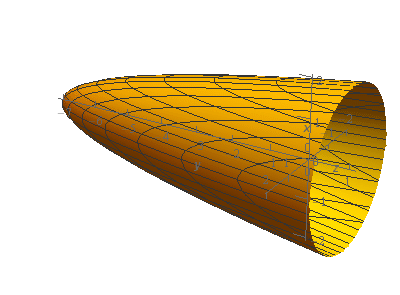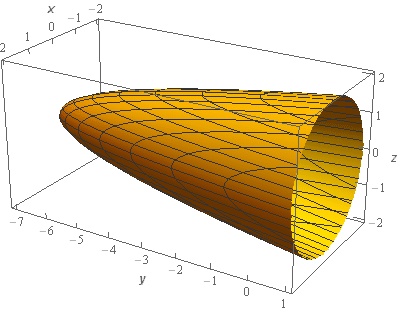Paul's Online Notes
Home / Calculus III / Multiple Integrals / Surface Area
Show Mobile Notice Show All Notes Hide All Notes
Mobile Notice
You appear to be on a device with a "narrow" screen width (i.e. you are probably on a mobile phone). Due to the nature of the mathematics on this site it is best views in landscape mode. If your device is not in landscape mode many of the equations will run off the side of your device (should be able to scroll to see them) and some of the menu items will be cut off due to the narrow screen width.

### Section 15.9 : Surface Area

4. Determine the surface area of the portion of $$y = 2{x^2} + 2{z^2} - 7$$that is inside the cylinder $${x^2} + {z^2} = 4$$.

Show All Steps Hide All Steps

Start Solution

Okay, let’s start off with a quick sketch of the surface so we can get a feel for what we’re dealing with.We’ve given the sketches with a set of “traditional” axes as well as a set of “box” axes to help visualize the surface.

So, we have an elliptic paraboloid centered on the $$y$$-axis. This also means that the region $$D$$ for our integral will be in the $$xz$$-plane and we’ll be needing polar coordinates for the integral.

Show Step 2

As noted above the region $$D$$ is going to be in the $$xz$$-plane and the surface is given in the form $$y = f\left( {x,z} \right)$$. The formula for surface area we gave in the notes is only for a region $$D$$ that is in the $$xy$$-plane with the surface given by $$z = f\left( {x,y} \right)$$ .

However, it shouldn’t be too difficult to see that all we need to do is modify the formula in the following manner to get one for this setup.

$S = \iint\limits_{D}{{\sqrt {{{\left[ {{f_x}} \right]}^2} + {{\left[ {{f_z}} \right]}^2} + 1} \,dA}}\hspace{0.25in}\,y = f\left( {x,z} \right)\hspace{0.25in}D\,\,{\mbox{is in the }}xz{\mbox{ - plane}}$

So, the integral for the surface area is,

$S = \iint\limits_{D}{{\sqrt {{{\left[ {4x} \right]}^2} + {{\left[ {4z} \right]}^2} + 1} \,dA}} = \iint\limits_{D}{{\sqrt {16{x^2} + 16{z^2} + 1} \,dA}}$ Show Step 3

Now, as we noted in Step 1 the region $$D$$ is in the $$xz$$-plane and because we are after the portion of the elliptical paraboloid that is inside the cylinder given by $${x^2} + {z^2} = 4$$ we can see that the region $$D$$ must therefore be the disk $${x^2} + {z^2} \le 4$$.

Also as noted in Step 1 we’ll be needing polar coordinates for this integral so here are the limits for the integral in terms of polar coordinates.

$\begin{array}{c}0 \le \theta \le 2\pi \\ 0 \le r \le 2\end{array}$ Show Step 4

Now, let’s convert the integral into polar coordinates. However, they won’t be the “standard” polar coordinates. Because $$D$$ is in the $$xz$$-plane we’ll need to use the following “modified” polar coordinates.

\begin{align*}x & = r\cos \theta \\ z & = r\sin \theta \\ {x^2} + {z^2} & = {r^2}\end{align*}

Converting the integral to polar coordinates then gives,

$S = \iint\limits_{D}{{\sqrt {16{x^2} + 16{z^2} + 1} \,dA}} = \int_{0}^{{2\pi }}{{\int_{0}^{2}{{r\sqrt {16{r^2} + 1} \,dr}}\,d\theta }}$

Don’t forget to pick up the extra $$r$$ from converting the $$dA$$ into polar coordinates. It is the same $$dA$$ as we use for the “standard” polar coordinates.

Show Step 5

Okay, all we need to do then is evaluate the integral.

\begin{align*}S & = \int_{0}^{{2\pi }}{{\int_{0}^{2}{{r\sqrt {16{r^2} + 1} \,dr}}\,d\theta }}\\ & = \int_{0}^{{2\pi }}{{\left. {\frac{1}{{48}}{{\left( {16{r^2} + 1} \right)}^{\frac{3}{2}}}} \right|_0^2\,d\theta }}\\ & = \int_{0}^{{2\pi }}{{\frac{1}{{48}}\left( {{{65}^{\frac{3}{2}}} - 1} \right)\,d\theta }}\\ & = \left. {\frac{1}{{48}}\left( {{{65}^{\frac{3}{2}}} - 1} \right)\theta } \right|_0^{2\pi } = \require{bbox} \bbox[2pt,border:1px solid black]{{\frac{\pi }{{24}}\left( {{{65}^{\frac{3}{2}}} - 1} \right) = 68.4667}}\end{align*}